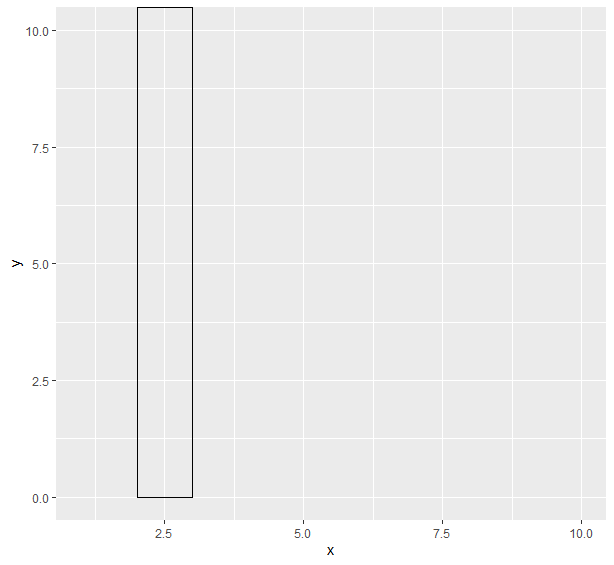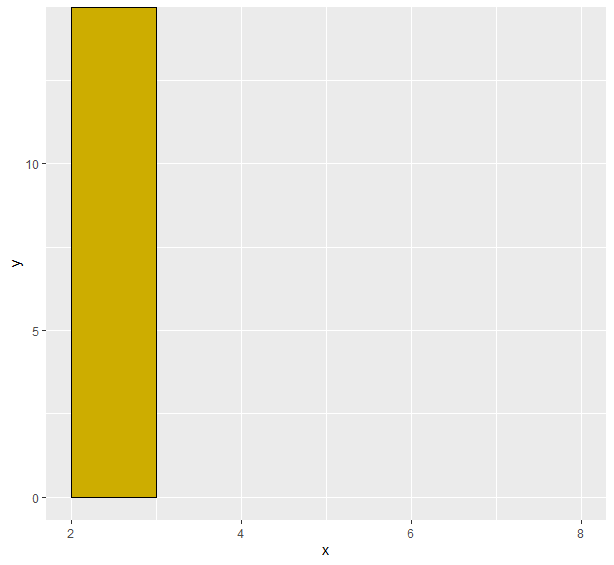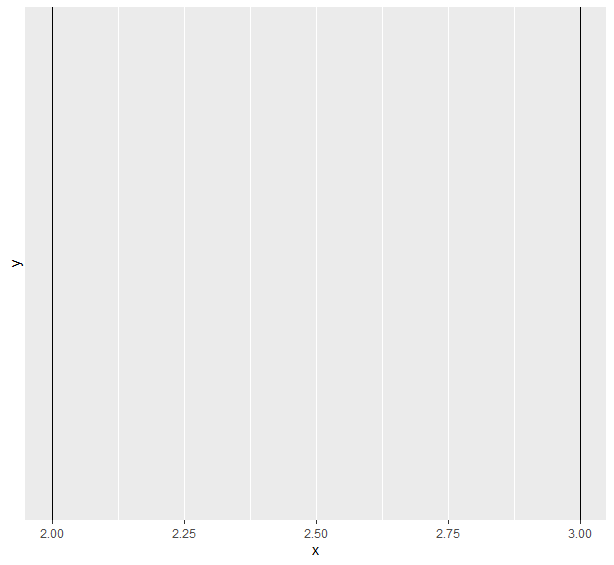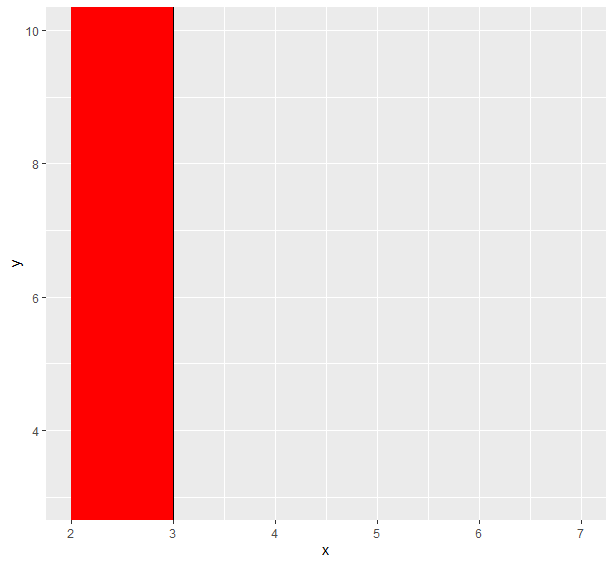# Plot Shaded Area between vertical lines in R

• Last Updated : 21 Aug, 2021

Plotting a shaded area between vertical lines means to put a vertical rectangle on a different color plot as compared to the rest of the plot area. We create this rectangle depending on the conditional values of the x-axis and represent the pivot area on the basis of characteristics of the data. This can be obtained using R and is described how in his article.

### Function used

• geom_rect() function is used to draw a rectangle

Syntax:

geom_rect(xmin, xmax, ymin, ymax, size, linetype, color, fill, alpha)

• geom_vline() draws a vertical line at a specified position

Syntax:

geom_vline(xintercept, size, linetype, color, alpha)

### Approach 1:

• Create frame
• Create plot
• Now draw a rectangle to represent two vertical lines- first we will draw a hollow rectangle
• Now add required color using fill parameter

Program 1: Draw two vertical files

## R

 `x<-``rpois``(10,5)` `y<-``rpois``(10,7)`   `df<-``data.frame``(x,y)`   `library``(ggplot2)` `ggplot``(df,``aes``(x,y))+` `  ``geom_rect``(``aes``(xmin=2,xmax=3,ymin=0,ymax=``Inf``),color=``"black"``,alpha=0)`

Output:Program 2: Fill color between lines

## R

 `x<-``rpois``(10,5)` `y<-``rpois``(10,7)`   `df<-``data.frame``(x,y)`   `library``(ggplot2)` `ggplot``(df,``aes``(x,y))+` `  ``geom_rect``(``aes``(xmin=2,xmax=3,ymin=0,ymax=``Inf``),color=``"black"``,alpha=0)`

Output:### Approach 2:

• Create frame
• Draw two vertical lines
• Fill color between them using a rectangle

Program 1: Drawing two lines

## R

 `x<-``rpois``(10,5)` `y<-``rpois``(10,7)`   `df<-``data.frame``(x,y)`   `library``(ggplot2)` `ggplot``(df,``aes``(x,y))+` `  ``geom_vline``(xintercept=2,color=``"black"``)+` `  ``geom_vline``(xintercept=3,color=``"black"``)`

Output:Program 2: Fill color between these lines

## R

 `x<-``rpois``(10,5)` `y<-``rpois``(10,7)`   `df<-``data.frame``(x,y)`   `library``(ggplot2)` `ggplot``(df,``aes``(x,y))+` `  ``geom_vline``(xintercept=2,color=``"black"``)+` `  ``geom_vline``(xintercept=3,color=``"black"``)+` `  ``geom_rect``(``aes``(xmin=2,xmax=3,ymin=-``Inf``,ymax=``Inf``),fill=``"red"``)`

Output:My Personal Notes arrow_drop_up
Recommended Articles
Page :